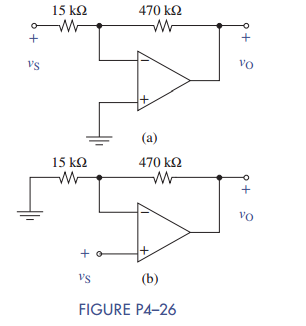Create an Account

Home / Questions / Find the voltage gain of each OP AMP circuit shown in Figure P4–26.

Find the voltage gain of each OP AMP circuit shown in Figure P4–26.

Find the voltage gain of each OP AMP circuit shown in Figure P4–26.Jul 28 2020 View more View LessSubscribe To Get Solution